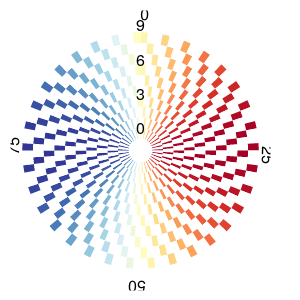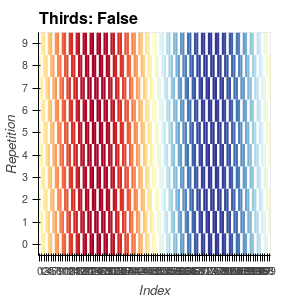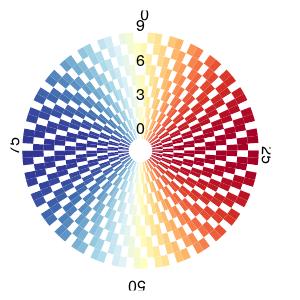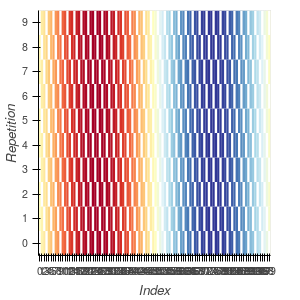{{ message }}

# Radial HeatMap option doesn't work with Dataset.to #2610

Closed
opened this issue Apr 23, 2018 · 3 comments
Closed

# Radial HeatMap option doesn't work with Dataset.to#2610

opened this issue Apr 23, 2018 · 3 comments
Labels
Milestone

###toddrjen commented Apr 23, 2018

 Radial HeatMaps work when declaring a HeatMap directly, but don't seem to work when using `Dataset.to`. Consider the following Dataset: ```import numpy as np import pandas as pd import holoviews as hv hv.extension('bokeh') x = np.arange(0, 1000) y = np.sin(x/1000*2*np.pi) index = x//10 rep = x%10 thirds = x%3==0 df = pd.DataFrame({"Value": y, "Index": index, "Repetition": rep, "Thirds": thirds}) ds = hv.Dataset(df, ['Repetition', 'Index', 'Thirds'], ['Value'])``` If we select one value of `Thirds` and make a radial HeatMap with that, it works properly: ```%opts HeatMap [radial=True] hv.HeatMap(ds.select(Thirds=True), ['Index', 'Repetition'])```However, if we use `Dataset.to` on the same data to get a dropdown, the HeatMap isn't radial: ```%opts HeatMap [radial=True] ds.to(hv.HeatMap, ['Index', 'Repetition'])```I would be willing to use an ugly workaround to get this working if it is available. The text was updated successfully, but these errors were encountered:
We are unable to convert the task to an issue at this time. Please try again.

###toddrjen commented Apr 24, 2018

 It looks like overlays don't work, either. Using the same Dataset as above, `ds`, this works: ```%opts HeatMap [radial=True] hm1 = hv.HeatMap(ds.select(Thirds=True), ['Index', 'Repetition']) hm1```And this works ```%opts HeatMap [radial=True] hm2 = hv.HeatMap(ds.select(Thirds=False), ['Index', 'Repetition']) hm2```And this works: ```%opts HeatMap [radial=True] hm1 + hm2``` But this doesn't: ```%opts HeatMap [radial=True] hm1*hm2```###toddrjen commented Apr 24, 2018

 There is also no colorbar with radial heatmaps: ```%%opts HeatMap [radial=True colorbar=True] hv.HeatMap(ds.select(Thirds=True), ['Index', 'Repetition'])``` Doesn't show any legend.

###philippjfr commented Apr 24, 2018

 Thanks, hopefully we can get a fix for this into 1.10.2.